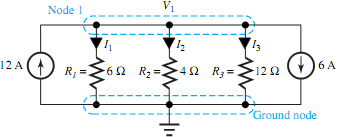## Show that conservation of power is satisfied by the circuit, Electrical Engineering

Assignment Help:

Given the network in Figure,

(a) Find the currents through resistors R1, R2, and R3.

(b) Compute the voltage V1.

(c) Show that the conservation of power is satisfied by the circuit.#### Operating system, what is serveces of operating system?

what is serveces of operating system?

#### 3rd year electrical/electronics project, I`m a 3rd year electrical engineer...

I`m a 3rd year electrical engineering student studying in Canada, and there is a new course they added in my university for 3rd year students called Engineering Project. from the

#### Factors which affect the inductance of an inductor, Factors which affect th...

Factors which affect the inductance of an inductor include:  i)  The number of turns of wire (N) - more turns the higher the inductance ii)  The cross-sectional area of the

#### Microcontroller, What are usue and all the details of 8051 microcontroller ...

What are usue and all the details of 8051 microcontroller with its block diagram pin diagram and all other details

Probelm: (a) Explain indexed addressing in 8051. (b) How does the 8051 distinguish between a byte address and a bit address? (c) How do open-loop and closed loop appli

example

#### Determine the transfer function, Q. For the networks shown in Figure, deter...

Q. For the networks shown in Figure, determine the transfer function G(s) = V o (s)/V i (s).

#### Where holes are majority carriers, Holes are majority carriers in (A) ...

Holes are majority carriers in (A) P-type semiconductors.  (B) N-type semiconductors. (C)  Insulators.            (D) Superconductors. Ans: Holes are m

#### Calculate rotor copper losses, A 20 h.p. , 3 phase, 400 volt, 60 hertz, 4 p...

A 20 h.p. , 3 phase, 400 volt, 60 hertz, 4 pole induction motor delivers full load at 5% slip. The mechanical rotational losses are 400W. Calculate (a) The electromagnetic torqu